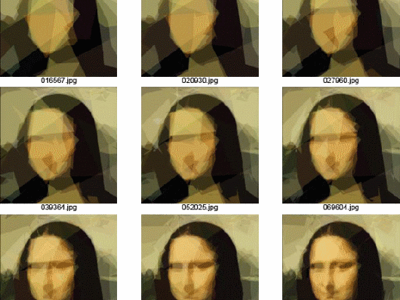# Genetic AlgorithmOne of the questions that has always been interested me in is how the process of “decision making” work. Us, humans, don’t act randomly and our decisions are based on a thought process inside our brains. What if a computer were to make a decision to get a certain result?

There are algorithms that evolve autonomously, called evolutionary algorithms.

The Genetic Algorithm (GA), is an example of them, that that evolves itself. The algorithm finds solutions to optimization and search problems. This evolutionary algorithm is used in large search spaces and inspired by natural selection. It is basically a trial and error process in which “the best” strategy” wins.

There are many amazing examples, here you can find two of them:

# Constrained OptimizationOptimization, a selection of best value for a set of parameters, is an important problem in different fields of science. When the best possible answer is subject to some constraints, then it is not an easy problem to deal with. However, if the constraint is an equality relationship, “Lagrange Multiplier” can be used to turn it into an unconstrained problem. This method is introduced byJoseph-Louis Lagrange about 200 years ago.

Let’s consider the problem of optimizing a function with no constraint. One would say take the derivative and find its roots to get the extrema of the function. Finding a closed form equation for the maxima or minima of the function is not trivial if the function should follow certain constraints. Instead, Lagrange introduced a method to turn the problem into an unconstrained one: Lagrange multipliers:

Goal: optimize f(x,y), subject to: g(x,y)-c=0

Define Lagrangian as:Consider contours of f which have a fixed f value while g(x,y)=c.

When the contour line of g intersects with f or cross the contour lines of f means while moving along the contour line the value of  f can change so it is not an extremum. While if the contour line of g meets contour lines of  f tangentially, the value of f is not changing and that point is a potential extremum. Mathematically it is equivalent to:Solving the Lagrange equation gives the optimized constrained values for f: